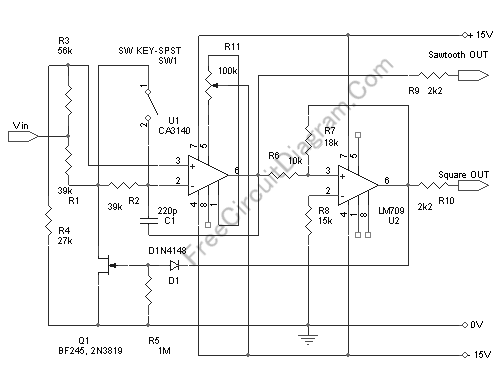## Simple Stand-Alone Voltage-to-Frequency Converter Using LM231/LM331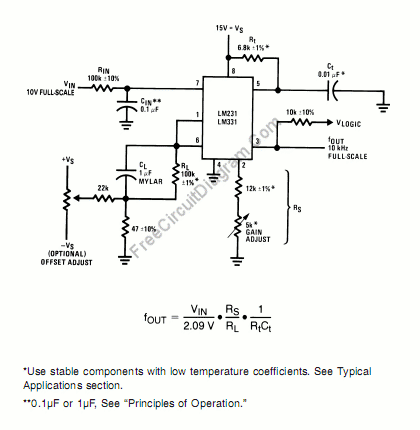Using LM231/331 chip, we can build a low-cost voltage-to-frequency converter, ideal for analog-to-digital conversion, frequency-to-voltage conversion, long-term integration, linear frequency modulation-demodulation, and many other functions.  When it’s used as voltage-to-frequency converter, the output will be a pulse train with frequency linearly equal to the applied input voltage. For higher level of accuracy against temperature drift, LM231A/LM331A can be used, let’s […]

## Wien Bridge Oscillator: The Circuit Schematic Diagram and The Design Formula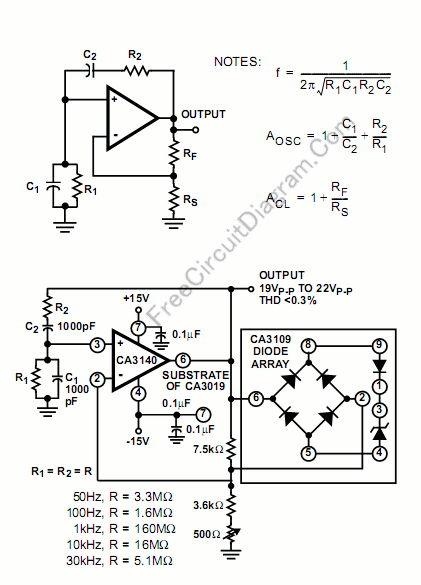The excellent high input impedance, high slew rate, and high voltage qualities of CA3140 op-amp make it suitable for Wien-bridge sine wave oscillator. The basic circuit for Wien-bridge sine wave oscillator is shown in the figure below (the upper part). You can see the formula in the figure, when R1=R2=R, and C1=C2=C, then the formula would be a very simple […]

## Square Oscillator Using Comparator: The Circuit Schematic Diagram and The Formula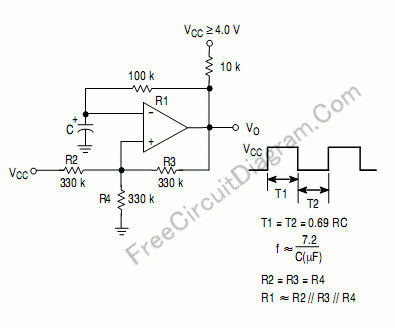Using a single supply quad comparator LM339, LM239, LM2901, LM2901V, NCV2901, or MC3302, we can build a square-wave oscillator with simple circuit and formula. Here is the schematic diagram: This circuit is suitable if you need  many square-wave oscillators in one circuit, since the quad packaged comparator provide four comparators in one single  integrated chip. [circuit’s schematic diagram source: Motorola […]

## Function Generator Using CA3080 and CA3130 Op-Amps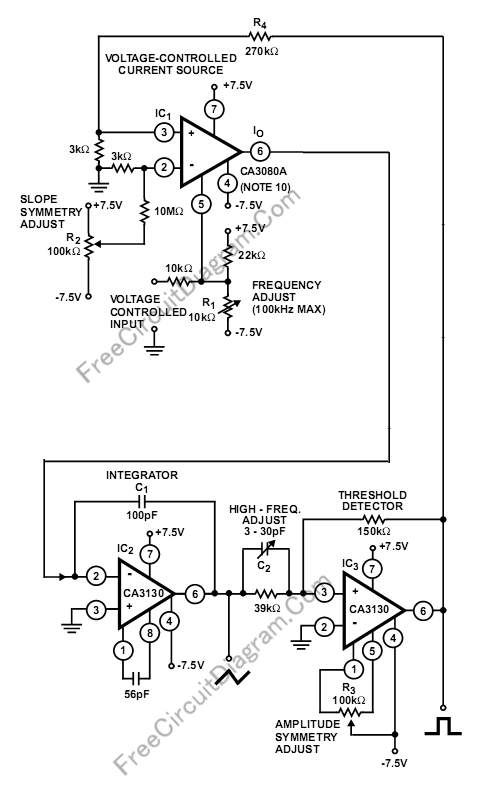The schematic diagram shown in the figure below show a function generator circuit using CA3130 in the integrator the threshold detection sections. Both triangular and square-wave signal are available from this circuit’s output, ranging from 0.1Hz to 100KHz (1:1000000 ratio). This frequency adjustment is done only by a single control R1. A voltage control input is available for externally-controlled frequency […]

## Pulse Generator (Oscillator) With Adjustable On-Off Period Using CA3130 Op-Amp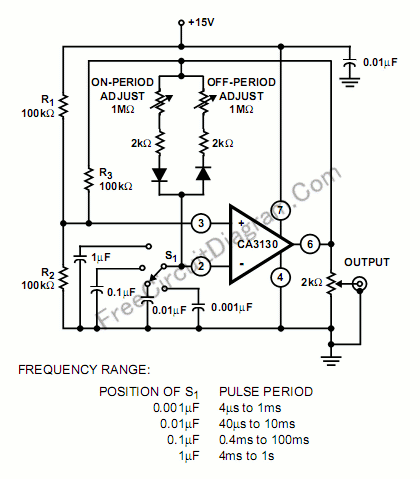One of the interesting features of CA3130 op-amp  is its high input resistance, because using such high  input resistance, we can build an astable multivibrator with high R/C ratios. The figure below shows the circuit’s schematic diagram, you can see the variable resistors to adjust the “on” and “off” periods independently. Resistors R1and R2 are used to bias the CA3130 […]